# Important Data Interpretation Set – 101

D.1 – 5 ) Study the following information carefully and answer the given questions

The following chart shows the breakup of students in five different colleges

Total number of students in college C is 4200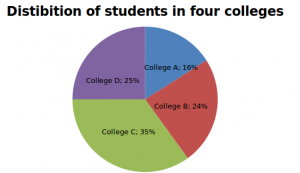Male to female ratio Male Female College A 11 13 College B 11 5 College C 2 5 College D 1 1

1) What is the total number of male students in colleges A and B together?

a) 2680

b) 2860

C) 2660

d) 3680

e) 2600

b) 2860

 Total number of students Total number of male students Total number of female students College A 1920 880 1040 College B 2880 1980 900 College C 4200 1200 3000 College D 3000 1500 1500

Total male students in colleges A and B=880+1980=2860

2) What is the difference between the total number of female students in college D and the total number of male students in college C?

a) 301

b) 310

c) 200

d) 300

e) 320

d) 300

 Total number of students Total number of male students Total number of female students College A 1920 880 1040 College B 2880 1980 900 College C 4200 1200 3000 College D 3000 1500 1500

Difference=1500-1200=300

3) What is the ratio between the total number of female students in College C and D together to the total number of students in colleges A and B together?

a) 11:12

b) 12:13

c) 16:15

d) 14:15

e) 15:16

e) 15:16

 Total number of students Total number of male students Total number of female students College A 1920 880 1040 College B 2880 1980 900 College C 4200 1200 3000 College D 3000 1500 1500

Ratio=(3000+1500):(1920+2880)=4500:4800=45:48=15:16

4) Total number of male students in all the colleges is what percent of the total number of students in College C and D together?

a) 74.23%

b) 78.99%

c) 77.22%

d) 89%

e) 87.22%

c) 77.22%

 Total number of students Total number of male students Total number of female students College A 1920 880 1040 College B 2880 1980 900 College C 4200 1200 3000 College D 3000 1500 1500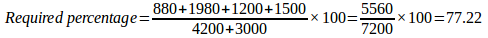5) In College E, total number of students equals to 95% of the total number of students in college D. Male to female ratio is 1:2. Then find the number of female students in College E

a) 2000

b) 1700

c) 1900

d) 1950

e) 2850

c) 1900

In college E total students=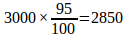Female students=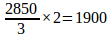### D 6 – 10 ) Study the following table carefully to answer that follow.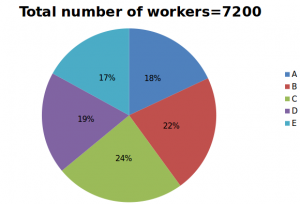M:F denotes ratio of male to female workers in each department

 DEPARTMENT M:F A 5:3 B 5:7 C 3:5 D 11:8 E 9:8

6) what is the total number of male workers and female workers working in department C and E respectively?

a) 684

b) 948

c) 1224

d) 1430

e) None of these

c) 1224

Total Number of workers in each department

A = 1296

B = 1584

C = 1728

D = 1368

E = 1224

 DEPARTMENT M:F A 810:486 B 660:924 C 648:1080 D 792:576 E 648:576

Total number of male workers in department C = 648

Total number of female workers in department E = 576

Total = 648 + 576 = 1224

7) what is the ratio of male workers in department A to female workers in department B?

a)153:154

b) 135:143

c) 135:137

d) 135:154

e) None of these

d) 135:154

Total Number of workers in each department

A = 1296

B = 1584

C = 1728

D = 1368

E = 1224

 DEPARTMENT M:F A 810:486 B 660:924 C 648:1080 D 792:576 E 648:576

Male workers in department A = 810

Female workers in department B = 924

810 : 924 = 405 : 462 = 135 : 154

8) Male workers of department A is what percent of the female workers in department C?

a) 55

b) 75

c) 65

d) 83

e) None of these

b) 75

Total Number of workers in each department

A = 1296

B = 1584

C = 1728

D = 1368

E = 1224

 DEPARTMENT M:F A 810:486 B 660:924 C 648:1080 D 792:576 E 648:576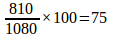9) Male workers of department B is what percent more than that of female workers in department D?

a) 14.58%

b) 18.45%

c) 25%

d) 32.25%

e) None of these

a) 14.58%

Total Number of workers in each department

A = 1296

B = 1584

C = 1728

D = 1368

E = 1224

 DEPARTMENT M:F A 810:486 B 660:924 C 648:1080 D 792:576 E 648:576

Male workers of department B = 660

Female workers of department D = 576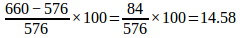10) What is the sum of average of male workers and average of female workers in all the departments?

a) 735

b) 811.6

c) 1321.5

d) 1440

e) None of these

d) 1440

Total Number of workers in each department

A = 1296

B = 1584

C = 1728

D = 1368

E = 1224

 DEPARTMENT M:F A 810:486 B 660:924 C 648:1080 D 792:576 E 648:576

Average of male workers = (810+660+648+792+648) = 3558/5= 711.6

Average of female workers = (486+924+1080+576+576) = 3642/5 = 728.4

Total Average = 711.6 + 728.4 = 1440